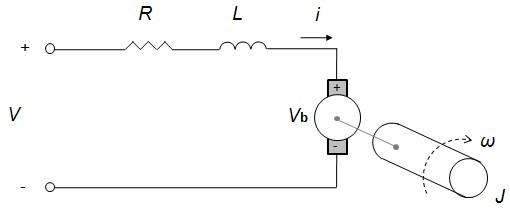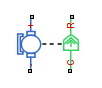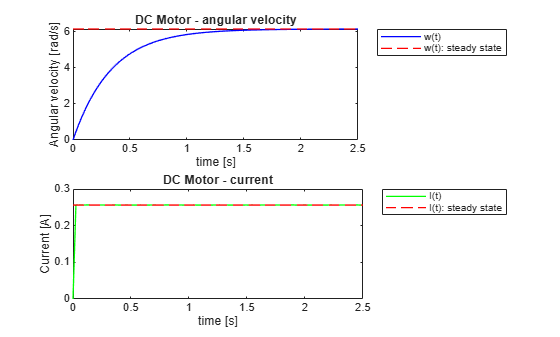Documentation

This is machine translation

Mouseover text to see original. Click the button below to return to the English version of the page.

Customize and Extend Simscape Libraries for a Custom DC Motor

Create a custom equation based components for the Simscape Library using the Symbolic Math Toolbox.

Introduction

The Symbolic Math Toolbox provides a flexible way to develop models from first engineering principles in any spatial dimension. You can develop mathematical models for steady state or transient physics.

You can develop and solve the equations required to represent the physics necessary for your component; and perform your own reduced-order model mapping between the inputs x and a quantity of interest f(x).

Here f is the custom component which can represent governing equations in the form of:

• Mathematical formulas

• Numerical simulations of ODEs and PDEs

The steps in this examples are

• Parameterizing a Simscape component using `symReadSSCVariables`

• Define custom equations for your Simscape component using `diff`

• Solve steady-state equations analytically using `solve` and `subs`

• Solve time-dependent equations numerically in MATLAB by using `matlabFunction` and `ode45`

• Create a Simscape component using `symWriteSSC`To run this example, you must have licenses for Simscape and Symbolic Math Toolbox.

DC Motor Model

A DC motor is a device for converting electrical energy into mechanical energy and vice versa. A schematic of a DC motor is shown below (left figure). Blocks that simulate DC motors are provided in Simscape Electrical™ (right figure), which is an optional product for Simscape.In this example, we will derive a reduced-order model representation of a DC Motor using the governing Ordinary Differential Equations (ODEs). For a DC motor, the electrical voltage and current is derived from Kirchhoff’s laws and the formula of the mechanical torque is derived from Newton’s laws. Using these equations we can implement a custom and parametric Simscape component.

Import Parameters and Variables of the Template Component

Suppose you have a Simscape component `MyMotorTemplate.ssc` in your current folder or on the default MATLAB path. This component has no equations yet. The template records the parameters and variables which will be used to develop our motor. You can use `type` to provide a preview of that template.

`type MyMotorTemplate.ssc`
```component MyMotorTemplate % Custom DC Motor % This block implements a custom DC motor nodes p = foundation.electrical.electrical; % +:left n = foundation.electrical.electrical; % -:left r = foundation.mechanical.rotational.rotational; % R:right c = foundation.mechanical.rotational.rotational; % C:right end parameters R = {3.9, 'Ohm'}; %Armature resistance L = {0.000012, 'H'}; %Armature inductance J = {0.000001, 'kg*m^2'}; %Inertia Dr = {0.000003, '(N*m*s)/rad'}; %Rotor damping Ki = {0.000072, '(N*m)/A'}; %Torque constant Kb = {0.000072, '(V*s)/rad'}; %Back-emf constant end variables torque = {0, 'N*m'}; %Total Torque tau = {0, 'N*m'}; %Electric Torque w = {0, 'rad/s'}; %Angular Velocity I = {0, 'A'}; %Current V = {0, 'V'}; %Applied voltage Vb = {0, 'V'}; %Counter electromotive force end function setup if(R<=0) error('Winding resistance must be greater than 0.'); end end branches torque : r.t -> c.t; % Through variable tau from r to c I : p.i -> n.i; % Through variable i from p to n end equations w == r.w -c.w; % Across variable w from r to c V == p.v -n.v; % Across variable v from p to n end end ```

Read the names, values, and units of the parameters from the template component.

`[parNames, parValues, parUnits] = symReadSSCParameters('MyMotorTemplate');`

Display the parameters, their values, and the corresponding units as vectors.

`vpa([parNames; parValues; parUnits],10)`
```ans =  $\left(\begin{array}{cccccc}\mathrm{Dr}& J& \mathrm{Kb}& \mathrm{Ki}& L& R\\ 0.000003& 0.000001& 0.000072& 0.000072& 0.000012& 3.9\\ 1 \frac{\mathrm{N}\mathrm{"newton - a physical unit of force."} \mathrm{m}\mathrm{"meter - a physical unit of length."} \mathrm{s}\mathrm{"second - a physical unit of time."}}{\mathrm{rad}\mathrm{"radian - a physical unit of plane angle."}}& 1 \mathrm{kg}\mathrm{"kilogram - a physical unit of mass."} {\mathrm{m}\mathrm{"meter - a physical unit of length."}}^{2}& 1 \frac{\mathrm{V}\mathrm{"volt - a physical unit of electric potential."} \mathrm{s}\mathrm{"second - a physical unit of time."}}{\mathrm{rad}\mathrm{"radian - a physical unit of plane angle."}}& 1 \frac{\mathrm{N}\mathrm{"newton - a physical unit of force."} \mathrm{m}\mathrm{"meter - a physical unit of length."}}{\mathrm{A}\mathrm{"ampere - a physical unit of electric current."}}& \mathrm{H}\mathrm{"henry - a physical unit of inductance."}& \mathrm{\Omega }\mathrm{"ohm - a physical unit of resistance."}\end{array}\right)$```

Add the parameter names to the MATLAB Workspace by using the `syms` function. Parameters appear as symbolic variables in the workspace. You can use `who` to list variables in the workspace.

```syms(parNames) syms```
```Your symbolic variables are: Dr J Kb Ki L R ans ```

Read and display the names of the component variables. Use `ReturnFunction` to simultaneously convert these variables to functions of the variable `t`.

```[varFuns, varValues, varUnits] = symReadSSCVariables('MyMotorTemplate', 'ReturnFunction', true); vpa([varFuns; varValues; varUnits],10)```
```ans =  $\left(\begin{array}{cccccc}\text{I}\left(t\right)& V\left(t\right)& \mathrm{Vb}\left(t\right)& \tau \left(t\right)& \mathrm{torque}\left(t\right)& w\left(t\right)\\ 0& 0& 0& 0& 0& 0\\ \mathrm{A}\mathrm{"ampere - a physical unit of electric current."}& \mathrm{V}\mathrm{"volt - a physical unit of electric potential."}& \mathrm{V}\mathrm{"volt - a physical unit of electric potential."}& 1 \mathrm{N}\mathrm{"newton - a physical unit of force."} \mathrm{m}\mathrm{"meter - a physical unit of length."}& 1 \mathrm{N}\mathrm{"newton - a physical unit of force."} \mathrm{m}\mathrm{"meter - a physical unit of length."}& 1 \frac{\mathrm{rad}\mathrm{"radian - a physical unit of plane angle."}}{\mathrm{s}\mathrm{"second - a physical unit of time."}}\end{array}\right)$```

Add the variable names to the MATLAB Workspace by using the `syms` function. Variables appear as symbolic functions in the workspace. Verify that you have declared all required symbolic variables and functions using `syms`.

```syms(varFuns) syms```
```Your symbolic variables are: Dr J Ki R Vb t torque I Kb L V ans tau w ```

Define the system of equations for modeling a DC Motor

The differential equations for the mechanical torque are defined as `eq1` and `eq2`. `I(t)`` `represents the current and `w(t)`` `the angular velocity`.`

`eq1 = torque + J*diff(w(t)) == -Dr*w(t) + tau(t)`
```eq1(t) =  ```
`eq2 = tau(t) == Ki*I(t)`
`eq2 = $\tau \left(t\right)=\mathrm{Ki} \text{I}\left(t\right)$`

The equations for the electrical voltage and current are `eq3` and `eq4`. V(t) and Vb(t) represent the applied voltage and counter electromotive force respectively.

`eq3 = L*diff(I(t)) + R*I(t) == V(t) - Vb(t)`
```eq3 =  ```
`eq4 = Vb(t) == Kb*w(t)`
`eq4 = $\mathrm{Vb}\left(t\right)=\mathrm{Kb} w\left(t\right)$`

We can list them together. Here, the motor’s torque is assumed to be proportional to the current.

`eqs = formula([eq1; eq2; eq3; eq4])`
```eqs =  ```

Extract the left and right sides of the equations.

```operands = children(eqs); operList = [ operands{:} ]; lhs = operList(1:2:end)```
```lhs =  ```
`rhs = operList(2:2:end)`
`rhs = $\left(\begin{array}{cccc}\tau \left(t\right)-\mathrm{Dr} w\left(t\right)& \mathrm{Ki} \text{I}\left(t\right)& V\left(t\right)-\mathrm{Vb}\left(t\right)& \mathrm{Kb} w\left(t\right)\end{array}\right)$`

The second and fourth equations define the values `tau(t)` and `Vb(t)`. To simplify the system of four equations to a system of two equations, substitute these values into the first and third equation.

`equations(1) = subs(eqs(1), lhs(2), rhs(2))`
```equations =  ```
`equations(2) = subs(eqs(3), lhs(4), rhs(4))`
```equations =  ```
`equations.'`
```ans =  ```

Before solving the equations, substitute parameters with their numeric values. Also, use `V(t) = 1`.

```equations = subs(equations, [parNames,V(t)], [parValues,1]); equations = subs(equations, torque, 0); vpa(equations.',10)```
```ans =  ```

Solve the equations for the steady state.

For this, remove the time dependency for the functions `w(t)` and `I(t)`. For example, substitute them with symbolic variables `ww` and `ii`.

```syms ww ii equations_steady = subs(equations, [w(t),I(t)], [ww,ii]); result = solve(equations_steady,ww,ii); steadyStateW = vpa(result.ww,10)```
`steadyStateW = $6.151120734$`
`steadyStateI = vpa(result.ii,10)`
`steadyStateI = $0.2562966973$`

Numerically simulate the symbolic expression in MATLAB by using `matlabFunction` and `ode45`.

Create a valid input for `ode45` from the symbolic equations. Use `odeToVectorField` to create the MATLAB procedure which represents the dynamical system $\frac{\mathrm{dY}}{\mathrm{dt}}=\mathit{f}\left(\mathit{t},\mathit{Y}\right)$ with the initial condition $\mathit{Y}\left({\mathit{t}}_{0}\right)={\mathit{Y}}_{0}$.

`[vfEquations, tVals] = odeToVectorField(equations)`
```vfEquations =  $\left(\begin{array}{c}\frac{147573952589676412928}{1770887431076117}-6 {Y}_{2}-\frac{2877692075498690052096 {Y}_{1}}{8854437155380585}\\ \frac{83010348331692984375 {Y}_{1}}{1152921504606846976}-\frac{27670116110564328125 {Y}_{2}}{9223372036854775808}\end{array}\right)$```
```tVals =  $\left(\begin{array}{c}\text{I}\\ w\end{array}\right)$```
`M = matlabFunction(vfEquations,'Vars', {'t','Y'})`
```M = function_handle with value: @(t,Y)[Y(1).*(-3.25e+5)-Y(2).*6.0+8.333333333333333e+4;Y(1).*7.2e+1-Y(2).*3.0] ```

Solve the differential equation using the initial conditions `w(0) = 0` and I`(0) = 0`.

`solution = ode45(M,[0 3],[0 0])`
```solution = struct with fields: solver: 'ode45' extdata: [1x1 struct] x: [1x293775 double] y: [2x293775 double] stats: [1x1 struct] idata: [1x1 struct] ```

Evaluate the solution at the following points in time t=[0.5,0.75,1]. The first value is the current `I(t)` and the second value is the angular velocity `w(t)`. We see that the solution for the angular velocity is starting to approach a steady state `steadyStateW`.

`deval(solution,0.5), deval(solution,.75), deval(solution,1)`
```ans = 2×1 0.2563 4.7795 ```
```ans = 2×1 0.2563 5.5034 ```
```ans = 2×1 0.2563 5.8453 ```
`steadyStateW`
`steadyStateW = $6.151120734$`

Plot the solution.

```time = linspace(0,2.5); iValues = deval(solution, time, 1); wValues = deval(solution, time, 2); steadyStateValuesI = vpa(steadyStateI*ones(1,100),10); steadyStateValuesW = vpa(steadyStateW*ones(1,100),10); figure; plot1 = subplot(2,1,1); plot2 = subplot(2,1,2); plot(plot1, time, wValues, 'blue', time, steadyStateValuesW, '--red', 'LineWidth', 1) plot(plot2, time, iValues, 'green', time, steadyStateValuesI, '--red', 'LineWidth', 1) title(plot1, 'DC Motor - angular velocity') title(plot2, 'DC Motor - current') ylabel(plot1, 'Angular velocity [rad/s]') ylabel(plot2, 'Current [A]') xlabel(plot1, 'time [s]') xlabel(plot2, 'time [s]') legend(plot1, 'w(t)', 'w(t): steady state', 'Location', 'northeastoutside') legend(plot2, 'I(t)', 'I(t): steady state', 'Location', 'northeastoutside')```Save your mathematical model back for use in Simscape.

Generate Simscape code using the original equations `eqs`.

```symWriteSSC('MyMotor.ssc', 'MyMotorTemplate.ssc', eqs, ... 'H1Header', '% Custom DC Motor', ... 'HelpText', {'% This block implements a custom DC motor'})```

Display the generated component by using the `type` command.

`type MyMotor.ssc`
```component MyMotor % Custom DC Motor % This block implements a custom DC motor nodes p = foundation.electrical.electrical; % +:left n = foundation.electrical.electrical; % -:left r = foundation.mechanical.rotational.rotational; % R:right c = foundation.mechanical.rotational.rotational; % C:right end parameters R = {3.9, 'Ohm'}; %Armature resistance L = {0.000012, 'H'}; %Armature inductance J = {0.000001, 'kg*m^2'}; %Inertia Dr = {0.000003, '(N*m*s)/rad'}; %Rotor damping Ki = {0.000072, '(N*m)/A'}; %Torque constant Kb = {0.000072, '(V*s)/rad'}; %Back-emf constant end variables torque = {0, 'N*m'}; %Total Torque tau = {0, 'N*m'}; %Electric Torque w = {0, 'rad/s'}; %Angular Velocity I = {0, 'A'}; %Current V = {0, 'V'}; %Applied voltage Vb = {0, 'V'}; %Counter electromotive force end function setup if(R<=0) error('Winding resistance must be greater than 0.'); end end branches torque : r.t -> c.t; % Through variable tau from r to c I : p.i -> n.i; % Through variable i from p to n end equations w == r.w -c.w; % Across variable w from r to c V == p.v -n.v; % Across variable v from p to n torque+J*w.der == tau-Dr*w; tau == Ki*I; L*I.der+R*I == V-Vb; Vb == Kb*w; end end ```

Build a Simscape library from the generated component.

```if ~isdir('+MyLib') mkdir +MyLib; end copyfile MyMotor.ssc +MyLib; ssc_build MyLib;```
```Generating Simulink library 'MyLib_lib' in the current directory '/tmp/Bdoc19a_1099451_254287/tpa0b9d9db/symbolic-ex98670381' ... ```

Mathematical Modeling with Symbolic Math Toolbox

Get examples and videos